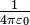# Planck units facts for kids

Kids Encyclopedia Facts

The Planck units are physical units of measurement. The system of these units was first developed by Max Planck. The definition of the five base Planck units are based only on five physical constants found in nature. When Planck units are used to express any of these five physical constants, the value is 1. This allows physicists to simplify many equations about physical law. Planck proposed these units in 1899. They are also known as natural units because the origin of their definition comes only from properties of nature and not from any human construct. Planck units are only one system of natural units among other systems. They are considered unique, because these units are not based on properties of any prototype object, or particle (that would be arbitrarily chosen) but are based only on properties of free space. The constants that Planck units, by definition, normalize to 1 are the:

• Gravitational constant, G;
• Reduced Planck constant, ħ;
• Speed of light in a vacuum, c;
• Coulomb constant,$\textstyle \frac{1}{4 \pi \varepsilon_0}$ (sometimes ke or k);
• Boltzmann's constant, kB (sometimes k).

Each of these constants can be associated with at least one fundamental physical theory: c with special relativity, G with general relativity and Newton's law of universal gravitation, ħ with quantum mechanics, ε0 with electrostatics, and kB with statistical mechanics and thermodynamics. Planck units are very important for theoretical physics, because they simplify several recurring algebraic expressions of physical law. They are particularly relevant in research on unified theories such as quantum gravity.

Planck units may sometimes be semi-humorously referred to by physicists as "God's units". They eliminate human centred arbitrariness from the system of units: some physicists argue that communication with extraterrestrial intelligence would have to use such a system of units to make common reference to scale. Unlike the meter and second, which exist as fundamental units in the SI system for historical reasons (in human history), the Planck length and Planck time are conceptually linked at a fundamental physical level.

Natural units help physicists to reframe questions. Frank Wilczek puts this succinctly:

...We see that the question [posed] is not, "Why is gravity so feeble?" but rather, "Why is the proton's mass so small?" For in Natural (Planck) Units, the strength of gravity simply is what it is, a primary quantity, while the proton's mass is the tiny number [1/(13 quintillion)]...

The strength of gravity is simply what it is and the strength of the electromagnetic force simply is what it is. The electromagnetic force operates on a different physical quantity (electric charge) than gravity (mass) so it cannot be compared directly to gravity. To note that gravity is an extremely weak force is, from the point-of-view of Planck units, like comparing apples and oranges. It is true that the electrostatic repulsive force between two protons (alone in free space) greatly exceeds the gravitational attractive force between the same two protons, and that is because the charge on the protons is approximately the Planck unit of charge but the mass of the protons is far, far less than the Planck mass.Planck units Facts for Kids. Kiddle Encyclopedia.